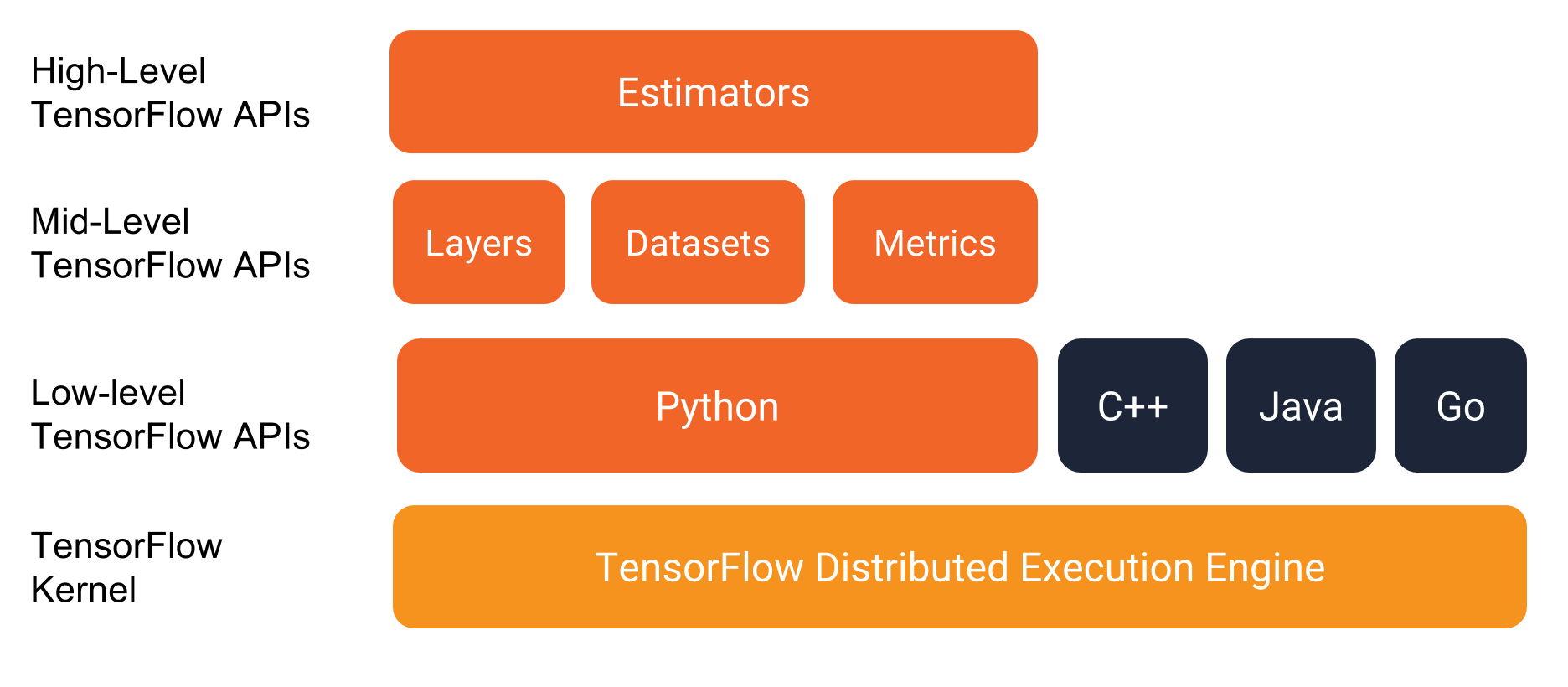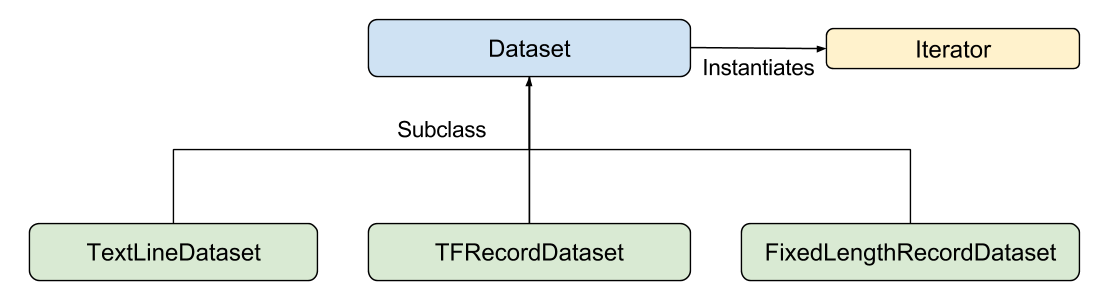TensorFlow is a machine learning framework that Google created and used to design, build, and train deep learning models. It supports complex and heavy numerical computations by using data flow graphs. This article is about summary and tips on TensorFlow.

# High Level APIs

## The Programming Stack

TensorFlow provides a programming stack consisting of multiple API layersThey recommend writing TensorFlow programs with the following APIs:

• Estimator: provides methods to train the model, to judge the model’s accuracy, and to generate predictions.
• “Estimator” is any class derived from tf.estimator.Estimator.
• TensorFlow provides a collection of pre-made Estimators (for example, LinearRegressor).
• Datasets: has methods to load and manipulate data, and feed it into your model. The datasets API meshes well with the Estimators API.
• An input function is a function that returns the following two-element tuple:
• “Features”: A Python dictionary in which each key is the name of a feature, each value is an array containing all of that features values.
• “Label”: An array containing the values of the label for every example.
• Simple demonstration of the format of the input function:
def input_evaluation_set():
features = { 'SepalLength': np.array([6.4, 5.0]),
'SepalWidth':  np.array([2.8, 2.3]),
'PetalLength': np.array([5.6, 3.3]),
'PetalWidth':  np.array([2.2, 1.0])}
labels = np.array([2, 1])
return features, labels

• Dataset API consists of the following classes:• Dataset: Base class containing methods to create and transform datasets. Also allows you to initialize a dataset from data in memory, or from a Python generator.
• TextLineDataset: Reads lines from text files.
• TFRecordDataset: Reads records from TFRecord files.
• FixedLengthRecordDataset: Reads fixed size records from binary files.
• Iterator: Provides a way to access one data set element at a time.
• Input pipeline example
def train_input_fn(features, labels, batch_size):
"""An input function for training"""
# Convert the inputs to a Dataset.
dataset = tf.data.Dataset.from_tensor_slices((dict(features), labels))

# Shuffle, repeat, and batch the examples.
dataset = dataset.shuffle(1000).repeat().batch(batch_size)

# Build the Iterator, and return the read end of the pipeline.
return dataset.make_one_shot_iterator().get_next()


• There are three other methods of getting data into a TensorFlow program:
• Feeding: Python code provides the data when running each step.
• Reading from files: an input pipeline reads the data from files at the beginning of a TensorFlow graph.
• Preloaded data: a constant or variable in the TensorFlow graph holds all the data (for small data sets).

## Data Types

• Tensor: tf.Tensor object represents the symbolic result of a TensorFlow operation. A tensor itself does not hold or store values in memory, but provides only an interface for retrieving the value referenced by the tensor.
• Variable: think of it as a normal variable which we use in programming languages. We initialize variables, we can modify it later as well.
• Variables can be described as persistent, mutable handles to in-memory buffers storing tensors. As such, variables are characterized by a certain shape and a fixed type.
• Placeholder: It doesn’t require initial value. Placeholder simply allocates block of memory for future use by using feed_dict and it has an unconstrained shape.

## Instantiate an Estimator

TensorFlow provides several pre-made classifier Estimators,

• tf.estimator.DNNClassifier — for deep models that perform multi-class classification.
• tf.estimator.DNNLinearCombinedClassifier — for wide-n-deep models.
• tf.estimator.LinearClassifier — for classifiers based on linear models.
# Build 2 hidden layer DNN with 10, 10 units respectively.
classifier = tf.estimator.DNNClassifier(
feature_columns=my_feature_columns,
# Two hidden layers of 10 nodes each.
hidden_units=[10, 10],
# The model must choose between 3 classes.
n_classes=3)


## Train the Model

Train the model by calling the Estimator’s train method as follows:

# Train the Model.
classifier.train(
input_fn=lambda:iris_data.train_input_fn(train_x, train_y, args.batch_size),
steps=args.train_steps)


## Evaluate the Trained Model

Evaluates the accuracy of the trained model on the test data:

# Evaluate the model.
eval_result = classifier.evaluate(
input_fn=lambda:iris_data.eval_input_fn(test_x, test_y, args.batch_size))

print('\nTest set accuracy: {accuracy:0.3f}\n'.format(**eval_result))


# Low Level APIs

TensorFlow Core programs consists of two discrete sections:

1. Building the computational graph (tf.Graph).
2. Running the computational graph (tf.Session).

## Tensor Values

• Tensor
• The central unit of data in TensorFlow.
• A tensor consists of a set of primitive values shaped into an array of any number of dimensions.
• A tensor’s rank is its number of dimensions.
• A tensor’s shape is a tuple of integers specifying the array’s length along each dimension.
• TensorFlow uses numpy arrays to represent tensor values.
3. # a rank 0 tensor; a scalar with shape [],
[1., 2., 3.] # a rank 1 tensor; a vector with shape 
[[1., 2., 3.], [4., 5., 6.]] # a rank 2 tensor; a matrix with shape [2, 3]
[[[1., 2., 3.]], [[7., 8., 9.]]] # a rank 3 tensor with shape [2, 1, 3]


## Graph

A computational graph is a series of TensorFlow operations arranged into a graph. The graph is composed of two types of objects.

1. Operations: The nodes of the graph, it describe calculations that consume and produce tensors.
2. Tensors: The edges in the graph, it represents the values that will flow through the graph and most TensorFlow functions return tf.Tensors.
• tf.Tensors: it does not have values, they are just handles to elements in the computation graph.

## Session

To evaluate tensors, instantiate a tf.Session object. A session encapsulates the state of the TensorFlow runtime, and runs TensorFlow operations. If a tf.Graph is like a .py file, a tf.Session is like the python executable.

sess = tf.Session() # Creates a tf.Session object
print (sess.run(total)) # run method to evaluate the total tensor


During a call to tf.Session.run any tf.Tensor only has a single value.

vec = tf.random_uniform(shape=(3,))
out1 = vec + 1
out2 = vec + 2
print(sess.run(vec))
print(sess.run(vec))
print(sess.run((out1, out2)))

""" Output"""
# The result shows a different random value on each call to run.
[ 0.52917576  0.64076328  0.68353939]
[ 0.66192627  0.89126778  0.06254101]
(
array([ 1.88408756,  1.87149239,  1.84057522], dtype=float32),
array([ 2.88408756,  2.87149239,  2.84057522], dtype=float32)
)


Some TensorFlow functions return tf.Operations instead of tf.Tensors. The result of calling run on an operation is None.

## TensorBoard

TensorBoard visualizes a computation graph. It can be done by following steps.

1. Save the computation graph to a TensorBoard summary file:
• writer = tf.summary.FileWriter('.')
• writer.add_graph(tf.get_default_graph())
2. This will produce an event file in the current directory with a name in the following format:
• events.out.tfevents.{timestamp}.{hostname}
3. In the terminal, launch TensorBoard:
• tensorboard --logdir

## Feeding

Placeholder provides a value later, like a function argument, so that a graph can be parameterized to accept external inputs.

"""Declare placeholder"""
x = tf.placeholder(tf.float32)
y = tf.placeholder(tf.float32)
z = x + y

"""Evaluate the graph with multiple inputs by using the feed_dict argument to feed concrete values to the placeholders"""
print(sess.run(z, feed_dict={x: 3, y: 4.5}))
print(sess.run(z, feed_dict={x: [1, 3], y: [2, 4]}))

"""Results"""
7.5
[ 3.  7.]

• feed_dict argument also can be used to overwrite any tensor in the graph.
• The only difference between placeholders and other tf.Tensors is that placeholders throw an error if no value is fed to them.

## Datasets

Placeholders work for simple experiments, but Datasets are the preferred method of streaming data into a model.

To get a runnable tf.Tensor from a Dataset, you must first convert it to a tf.data.Iterator, and then call the Iterator’s get_next method. The simplest way to create an Iterator is with the make_one_shot_iterator method.

"""next_item tensor will return a rwo from the my_data array on each run call"""
my_data = [
[0, 1,],
[2, 3,],
[4, 5,],
[6, 7,],
]
slices = tf.data.Dataset.from_tensor_slices(my_data)
next_item = slices.make_one_shot_iterator().get_next()


Reaching the end of the data stream causes Dataset to throw an OurOfRangeError.

## Layers

Layers is a way to add trainable parameters to a graph. Layers package together both the variables and the operations that act on them. For example, a densely-connected layer performs a weighted sum across all inputs for each output and applies an optional activation function. The connection weights and biases are managed by the layer object.

• Creating Layers
• To apply a layer to an input, call the layer as if it were a function.
x = tf.placeholder(tf.float32, shape=[None, 3])
linear_model = tf.layers.Dense(units=1)
y = linear_model(x)

• Initializing Layers
• The layer contains variables that must be initialized before they can be used.
# tf.global_variables_initializer op will initialize all the global variables when we run it with tf.Session.run
init = tf.global_variables_initializer()
sess.run(init)

• Executing Layers
• Evaluate linear_model’s output tensor as we would any other tensor.
print(sess.run(y, {x: [[1, 2, 3],[4, 5, 6]]}))

• Layer Function Shortcuts
• For each layer class (like tf.layers.Dense) TensorFlow also supplies a shortcut function (like tf.layers.dense).
• The only difference is that the shortcut function versions create and run the layer in a single call.
• It does not allow access to the tf.layers.Layer object which makes introspection and debugging more difficult, and layer reuse impossible.
x = tf.placeholder(tf.float32, shape=[None, 3])
y = tf.layers.dense(x, units=1)

init = tf.global_variables_initializer()
sess.run(init)

print(sess.run(y, {x: [[1, 2, 3], [4, 5, 6]]}))


## Feature Clumns

The easiest way to experiment with feature columns is using the tf.feature_column.input_layer function. This function only accepts dense columns as inputs, so to view the result of a categorical column you must wrap it in an tf.feature_column.indicator_column.

features = {
'sales' : [, , , ],
'department': ['sports', 'sports', 'gardening', 'gardening']}

department_column = tf.feature_column.categorical_column_with_vocabulary_list(
'department', ['sports', 'gardening'])
department_column = tf.feature_column.indicator_column(department_column)

columns = [
tf.feature_column.numeric_column('sales'),
department_column
]

inputs = tf.feature_column.input_layer(features, columns)


Running the inputs tensor will parse the features into a batch of vectors. Feature columns can have internal state, like layers, so they often need to be initialized. Categorical columns use lookup tables internally and these require a separate initialization op, tf.tables_initializer.

"""Initialize feature columns"""
var_init = tf.global_variables_initializer()
table_init = tf.tables_initializer()
sess = tf.Session()
sess.run((var_init, table_init))

"""print the output"""
print(sess.run(inputs))

# The feature columns have packed the input vectors with the one-hot "department" as the first two indices and "sales" as the third.
[[  1.   0.   5.]
[  1.   0.  10.]
[  0.   1.   8.]
[  0.   1.   9.]]


## Training

"""1. Define the data"""
x = tf.constant([, , , ], dtype=tf.float32)
y_true = tf.constant([, [-1], [-2], [-3]], dtype=tf.float32)

"""2. Define the model"""
linear_model = tf.layers.Dense(units=1)
y_pred = linear_model(x)

"""3. Define the loss"""
loss = tf.losses.mean_squared_error(labels=y_true, predictions=y_pred)

"""4. Define the optimizer"""